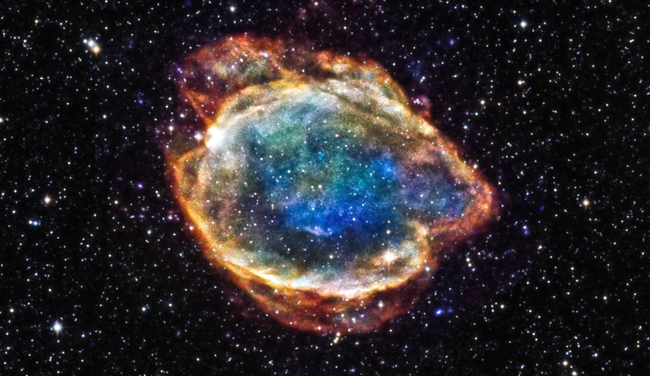# Implementing a Star Camera

The orbit of a satellite can be computed from it’s location and velocity. What is more challenging is accurately determining its orientation. This is really important because satellites normally need to orient themselves to maximize the sunlight on their solar arrays and also point camera and other sensors in specific directions. There isn’t a lot to navigate with in space except the billions and billions of stars which is what a star camera does.

I thought it would be fun to try and implement a star camera. Let’s say we are given a picture of the night sky and need to determine which direction the camera is pointing in. To do this we need to compute a `direction` vector and also an `up` vector orthogonal to it so we know what the orientation of the camera is. For example, here is an image taken of Sirius, the brightest star in the sky. The un-cropped image in `1000` pixels square and the field of view is `20` degrees.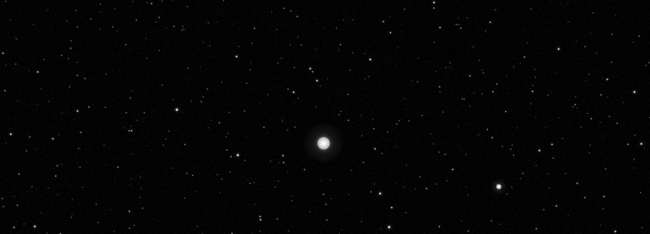To work out which direction our camera is pointing we also need some kind of map of the stars. One such map was generated from the Hipparcos satellite which was launched in 1989. It was the first space experiment devoted to precision astrometry. Hipparcos spent the next 4 years creeping around the solar system looking at things and the resulting Hipparcos Catalogue - a high-precision catalogue of more than 118,200 stars - was published in 1997. It never reached New York Times Bestseller’s list but it’s still a great read. If we look at the Hipparcos Catalogue we see that each star has four number associated with it. Here’s an extract:

``````108644  5.7622335593    -0.0478353703   9.5057
108647  5.7623641166    -0.2399106245   7.6062
108649  5.7624094330    -0.2472136702   7.8371
108651  5.7624495466    -0.8732797806   9.4559
108656  5.7626566242    -0.4066098406   7.9346
108658  5.7627242328    -0.5848303489   8.8601
``````

The first number is an `id` of the star. The second and third numbers are the `right ascension` and `declination` of the star. These can be thought of as a projection of the Earth bound longitude and latitude system to the sky, respectively. The forth number is the apparent magnitude of the star. Which is how shiny it is. It’s a negative logarithmic measure of its brightness - so the smaller the number the brighter the star. The first thing we need to do is pick out the brightest stars in the image. We can use a blob detector for this:

``````import cv2

def process_image(filename):

# threshold the image to black and white
ret, sky = cv2.threshold(sky,127,255,cv2.THRESH_BINARY)
sky = 255 - sky

params = cv2.SimpleBlobDetector_Params()

# detection thresholds
params.filterByArea = True
params.minArea = 10

# Set up the detector with parameters.
detector = cv2.SimpleBlobDetector(params)

# Detect blobs.
keypoints = detector.detect(sky)

return keypoints
``````

This works well ands pick out the brightest stars in the image.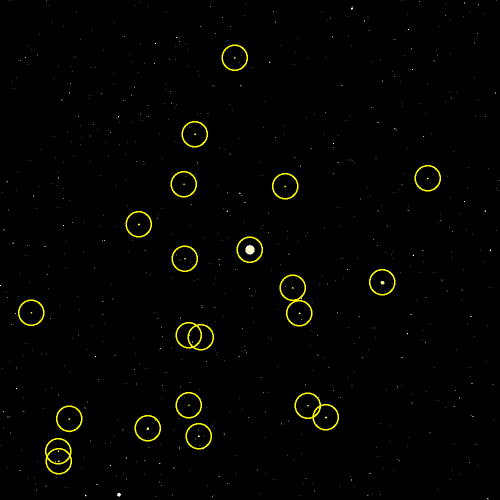Next we need to to convert the locations of the stars in the image to image coordinates. Since we know the size of the image and the field of view we can compute the focal length and convert the pixel coordinates to image coordinates:

``````import numpy as np

FOV  = 20
SIZE = 1000

def get_dir(x, y):

focal = 0.5 * SIZE / np.tan(05 * FOV * np.pi / 180.0)
v = np.array([x - 499.5, 499.5 - y, -focal])
v = s / np.linalg.norm(v)
return s
``````

From this we can compute the angle between any two stars in the image with:

``````def angle_between(v1, v2):
v1 = v1 / np.linalg.norm(v1)
v2 = v2 / np.linalg.norm(v2)

dot = np.dot(v1, v2)
dot = min(max(dot, -1.0), 1.0);

return np.arccos(dot)
``````

Now let’s turn to the catalogue of stars. We need to match these blobs to stars in the catalogue.We can convert right ascension and declination to a unit vector by converting from spherical to cartesian coordinates:

``````from numpy import cos, sin

def xyz(ra, dec):
x = cos(ra) * cos(dec)
y = sin(ra) * cos(dec)
z = sin(dec)

v = np.array([x, y, z])
v = v / np.linalg.norm(v)

return v
``````

Now we need to find a set of stars in the image and a corresponding set of stars in the catalogue where the angles between any pairs of stars are the same this will tell us which stars we are looking at and we can use their coordinates to reconstruct the `direction` and `up` vectors of where we are looking. We can do this by assigning a random star from the catalogue to the first blob. Then we keep assigning stars to blobs but each time we ensure that the distance between the stars in the set is almost equal to the distance betweens the blobs. We recursively assign stars and as we add more set becomes more consistent. I used `6` stars in the matching and for the image at the top we match a number of stars near a region of sky called the Winter Hexagon.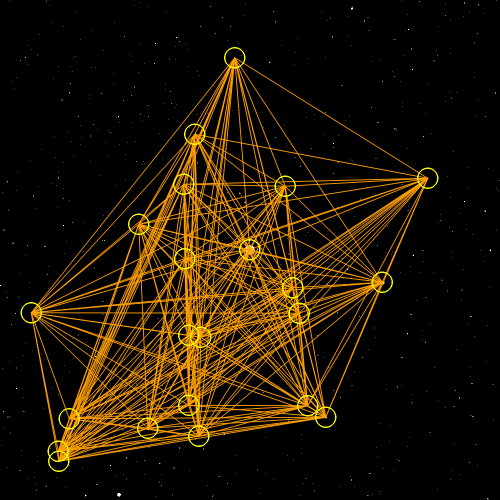Using this we can compute the transform from image to star coordinates:

``````def find_transform(stars, blobs):

v1 = stars
v2 = stars
v3 = stars

k1 = blobs
k2 = blobs
k3 = blobs

star_directions = np.vstack([v1, v2, v3]).T
image_directions = np.vstack([k1, k2, k3]).T

inv_star_directions = np.linalg.inv(star_directions)
transform = image_directions.dot(inv_star_directions)

direction = -transform[2, :]
up = transform[1, :]

return xfm, dir, up
``````

Which gives us the correct `direction` and `up` vector pointing towards Sirius.

``````-0.18748276  0.93921052 -0.28763485
-0.05714072  0.28195542  0.95772443
``````

Let’s try another image. This is of the North sky.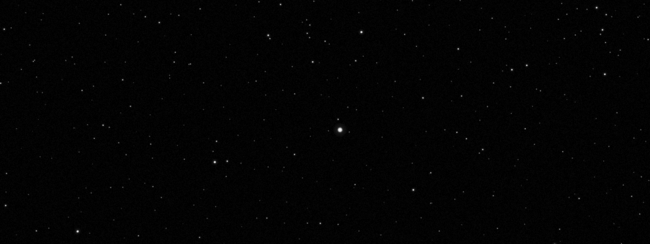Running the same code and out pops the correct vector pointing North.

``````0 0 1
1 0 0
``````

I’m sure the implementation used in satellite and ship navigation system are more involved, but this was fun to implement. Here is a link to the complete code. Interestingly, Hipparcos’s successor, Gaia was launched on 19 December 2013 with the intention of constructing a 3D space catalog of approximately 1 billion astronomical objects. The first catalogue of stars will be released later this year.